xNegative massEncyclopedia
In theoretical physics
Theoretical physics
Theoretical physics is a branch of physics which employs mathematical models and abstractions of physics to rationalize, explain and predict natural phenomena...

, negative mass is a hypothetical concept of matter whose mass
Mass
Mass can be defined as a quantitive measure of the resistance an object has to change in its velocity.In physics, mass commonly refers to any of the following three properties of matter, which have been shown experimentally to be equivalent:...

is of opposite sign to the mass of the normal matter. Such matter would violate one or more energy condition
Energy condition
In relativistic classical field theories of gravitation, particularly general relativity, an energy condition is one of various alternative conditions which can be applied to the matter content of the theory, when it is either not possible or desirable to specify this content explicitly...

s and show some strange properties such as being repelled rather than attracted by gravity. It is used in certain speculative theories, such as on the construction of wormhole
Wormhole
In physics, a wormhole is a hypothetical topological feature of spacetime that would be, fundamentally, a "shortcut" through spacetime. For a simple visual explanation of a wormhole, consider spacetime visualized as a two-dimensional surface. If this surface is folded along a third dimension, it...

s. The closest known real representative of such exotic matter is a region of pseudo-negative pressure
Negative pressure
Negative pressure may refer to:*negative pressure as opposed to positive pressure*stretched liquid*vacuum*negative gauge pressure, a way of expressing pressure measurements below atmospheric pressure*suction*transpirational pull...

density produced by the Casimir effect
Casimir effect
In quantum field theory, the Casimir effect and the Casimir–Polder force are physical forces arising from a quantized field. The typical example is of two uncharged metallic plates in a vacuum, like capacitors placed a few micrometers apart, without any external electromagnetic field...

.

## Inertial versus gravitational

Ever since Newton
Isaac Newton
Sir Isaac Newton PRS was an English physicist, mathematician, astronomer, natural philosopher, alchemist, and theologian, who has been "considered by many to be the greatest and most influential scientist who ever lived."...

first formulated his theory of gravity, there have been at least three conceptually distinct quantities called mass
Mass
Mass can be defined as a quantitive measure of the resistance an object has to change in its velocity.In physics, mass commonly refers to any of the following three properties of matter, which have been shown experimentally to be equivalent:...

: inertial mass, "active" gravitational mass (that is, the source of the gravitational field), and "passive" gravitational mass (that is, the mass that is evident from the force produced in a gravitational field). The Einstein equivalence principle
Equivalence principle
In the physics of general relativity, the equivalence principle is any of several related concepts dealing with the equivalence of gravitational and inertial mass, and to Albert Einstein's assertion that the gravitational "force" as experienced locally while standing on a massive body is actually...

postulates that inertial mass must equal passive gravitational mass; while the law of conservation of momentum
Momentum
In classical mechanics, linear momentum or translational momentum is the product of the mass and velocity of an object...

requires that active and passive gravitational mass must be identical. All experimental evidence to date has found these are indeed always the same. In considering hypothetical particles with negative mass, it is important to consider which of these concepts of mass are negative; however, in most analyses of negative mass, it is assumed that the equivalence principle and conservation of momentum continue to apply.

In 1957, Hermann Bondi
Hermann Bondi
Sir Hermann Bondi, KCB, FRS was an Anglo-Austrian mathematician and cosmologist. He is best known for developing the steady-state theory of the universe with Fred Hoyle and Thomas Gold as an alternative to the Big Bang theory, but his most lasting legacy will probably be his important...

suggested in a paper in Reviews of Modern Physics
Reviews of Modern Physics
The Reviews of Modern Physics is a journal of the American Physical Society. The journal started in paper form. All volumes are also online by subscription.Issue 1, Volume 1 consisted of the review by...

that mass might be negative as well as positive. He pointed out that this does not entail a logical contradiction, as long as all three forms of mass are negative, but that the assumption of negative mass involves some counter-intuitive form of motion.

From Newton's second law
Classical mechanics
In physics, classical mechanics is one of the two major sub-fields of mechanics, which is concerned with the set of physical laws describing the motion of bodies under the action of a system of forces...

: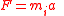Thus it can be seen that an object with negative inertial mass would be expected to accelerate in the opposite direction to that in which it was pushed, which is arguably a strange concept. If the "push" is based on the electromagnetic force, this would simply mean the mass accelerates in the opposite direction than what one would expect based on its charge; for example, an object with negative inertial mass and positive charge would accelerate away from objects with positive mass and negative charge, and accelerate towards objects with positive mass and positive charge, the opposite of the normal rule that like charges repel and opposite charges attract.

If one were to treat inertial mass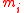, passive gravitational mass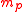, and active gravitational mass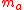distinctly, then Newton's law of universal gravitation would take the form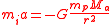(where a is the acceleration of an object with inertial massand passive gravitational massin the gravitational field generated by a different object with active gravitational mass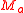, with r as the distance between the two objects and G as the gravitational constant
Gravitational constant
The gravitational constant, denoted G, is an empirical physical constant involved in the calculation of the gravitational attraction between objects with mass. It appears in Newton's law of universal gravitation and in Einstein's theory of general relativity. It is also known as the universal...

)

Thus objects with negative passive gravitational mass, but with positive inertial mass, would be expected to be repelled by positive active masses, and attracted to negative active masses. However, any difference between inertial and gravitational mass would violate the equivalence principle
Equivalence principle
In the physics of general relativity, the equivalence principle is any of several related concepts dealing with the equivalence of gravitational and inertial mass, and to Albert Einstein's assertion that the gravitational "force" as experienced locally while standing on a massive body is actually...

of general relativity
General relativity
General relativity or the general theory of relativity is the geometric theory of gravitation published by Albert Einstein in 1916. It is the current description of gravitation in modern physics...

. For an object where both the inertial and gravitational masses were negative and equal, we could cancel outandfrom the equation, and conclude that its acceleration a in the gravitational field from a body with positive active gravitational mass (say, the planet Earth) would be no different from the acceleration of an object with positive passive gravitational and inertial mass (so a small negative mass object would fall towards the Earth at the same rate as any other object).

On the other hand, if we have a small object with equal inertial and passive gravitational masses falling in the gravitational field of an object with negative active gravitational mass (a small mass dropped above a negative-mass planet, say), then canceling outandwould indicate that the acceleration a of the small object is proportional to the negative active gravitational massof the object creating the gravitational field, so the small object would actually accelerate away from the negative-mass object rather than towards it (and this is true regardless of whether the small object's inertial and passive gravitational masses were both positive or both negative). So, as long as inertial mass and gravitational mass are always equal as required by the equivalence principle, positive active gravitational mass would be universally attractive (both negative-mass and positive-mass objects would be pulled towards an object with positive active gravitational mass), while negative active gravitational mass would be universally repulsive (both negative-mass and positive-mass objects would be pushed away).

## Forward's analysis

Although no particles are known to have negative mass, physicists (primarily Hermann Bondi
Hermann Bondi
Sir Hermann Bondi, KCB, FRS was an Anglo-Austrian mathematician and cosmologist. He is best known for developing the steady-state theory of the universe with Fred Hoyle and Thomas Gold as an alternative to the Big Bang theory, but his most lasting legacy will probably be his important...

and Robert L. Forward) have been able to describe some of the anticipated properties such particles may have. Assuming that all three concepts of mass are equivalent it would produce a system where negative masses are attracted to positive masses, yet positive masses are repelled away from negative masses. Negative masses would produce an attractive force on one another, but would move apart because of their negative inertial masses.

For a negative value ofwith positive value of,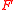is negative (repulsive); thus it would appear that a negative mass would accelerate away from a positive mass. But because such an object would also possess negative inertial mass it would accelerate in the opposite direction to. As Bondi pointed out, it can be shown that if both masses are of equal but opposite mass, the combined system of positive and negative particles will accelerate indefinitely without any additional input into the system.

This behavior is completely inconsistent with a common-sense approach and the expected behaviour of 'normal' matter; but is completely mathematically consistent and introduces no violation of conservation of momentum or energy
Conservation of energy
The nineteenth century law of conservation of energy is a law of physics. It states that the total amount of energy in an isolated system remains constant over time. The total energy is said to be conserved over time...

. If the masses are equal in magnitude but opposite in sign, then the momentum of the system remains zero if they both travel together and accelerate together, no matter what their speed: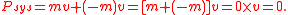And equivalently for the kinetic energy
Kinetic energy
The kinetic energy of an object is the energy which it possesses due to its motion.It is defined as the work needed to accelerate a body of a given mass from rest to its stated velocity. Having gained this energy during its acceleration, the body maintains this kinetic energy unless its speed changes...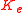: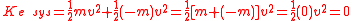Forward extended Bondi's analysis to additional cases, and showed that even if the two masses m(-) and m(+) are not the same, the conservation laws remain unbroken.

This behaviour can produce bizarre results: for instance, a gas containing a mixture of positive and negative matter particles will have the positive matter portion increase in temperature
Temperature
Temperature is a physical property of matter that quantitatively expresses the common notions of hot and cold. Objects of low temperature are cold, while various degrees of higher temperatures are referred to as warm or hot...

without bound. However, the negative matter portion gains negative temperature at the same rate, again balancing out. Geoffrey A. Landis
Geoffrey A. Landis
Geoffrey A. Landis is an American scientist, working for the National Aeronautics and Space Administration on planetary exploration, interstellar propulsion, solar power and photovoltaics...

pointed out other implications of Forward's analysis, including noting that although negative mass particles would repel each other gravitationally, the electrostatic force would be attractive for like-charges
Charge (physics)
In physics, a charge may refer to one of many different quantities, such as the electric charge in electromagnetism or the color charge in quantum chromodynamics. Charges are associated with conserved quantum numbers.-Formal definition:...

and repulsive for opposite charges.

Forward used the properties of negative-mass matter to create the diametric drive, a design for spacecraft propulsion
Spacecraft propulsion
Spacecraft propulsion is any method used to accelerate spacecraft and artificial satellites. There are many different methods. Each method has drawbacks and advantages, and spacecraft propulsion is an active area of research. However, most spacecraft today are propelled by forcing a gas from the...

using negative mass that requires no energy input and no reaction mass
Working mass
Working mass is a mass against which a system operates in order to produce acceleration.In the case of a rocket, for example, the reaction mass is the fuel shot backwards to provide propulsion. All acceleration requires an exchange of momentum, which can be thought of as the "unit of movement"...

to achieve arbitrarily high acceleration.

Forward also coined a term, "nullification" to describe what happens when ordinary matter and negative matter meet: they are expected to be able to "cancel-out" or "nullify" each other's existence. An interaction between equal quantities of positive and negative mass matter would release no energy, but since the only configuration of such particles which has zero momentum (both particles moving with the same velocity in the same direction) does not produce a collision, all such interactions would leave a surplus of momentum, which is classically forbidden.

## In general relativity

In general relativity
General relativity
General relativity or the general theory of relativity is the geometric theory of gravitation published by Albert Einstein in 1916. It is the current description of gravitation in modern physics...

, negative mass is generalized to refer to any region of space in which for some observers the mass density is measured to be negative. This can occur due to negative mass, or could be a region of space in which the stress component of the Einstein stress-energy tensor
Stress-energy tensor
The stress–energy tensor is a tensor quantity in physics that describes the density and flux of energy and momentum in spacetime, generalizing the stress tensor of Newtonian physics. It is an attribute of matter, radiation, and non-gravitational force fields...

is larger in magnitude than the mass density. All of these are violations of one or another variant of the positive energy condition
Energy condition
In relativistic classical field theories of gravitation, particularly general relativity, an energy condition is one of various alternative conditions which can be applied to the matter content of the theory, when it is either not possible or desirable to specify this content explicitly...

of Einstein's general theory of relativity; however, the positive energy condition is not a required condition for the mathematical consistency of the theory. (Various versions of the positive energy condition, weak energy condition, dominant energy condition, etc., are discussed in mathematical detail by Visser.)

Morris, Thorne
Kip Thorne
Kip Stephen Thorne is an American theoretical physicist, known for his prolific contributions in gravitation physics and astrophysics and for having trained a generation of scientists...

and Yurtsever pointed out that the quantum mechanics of the Casimir effect
Casimir effect
In quantum field theory, the Casimir effect and the Casimir–Polder force are physical forces arising from a quantized field. The typical example is of two uncharged metallic plates in a vacuum, like capacitors placed a few micrometers apart, without any external electromagnetic field...

can be used to produce a locally mass-negative region of space-time. In this article, and subsequent work by others, they showed that negative matter could be used to stabilize a wormhole
Wormhole
In physics, a wormhole is a hypothetical topological feature of spacetime that would be, fundamentally, a "shortcut" through spacetime. For a simple visual explanation of a wormhole, consider spacetime visualized as a two-dimensional surface. If this surface is folded along a third dimension, it...

. Cramer et al. argue that such wormholes might have been created in the early universe, stabilized by negative-mass loops of cosmic string
Cosmic string
Cosmic strings are hypothetical 1-dimensional topological defects which may have formed during a symmetry breaking phase transition in the early universe when the topology of the vacuum manifold associated to this symmetry breaking is not simply connected. It is expected that at least one string...

. Stephen Hawking
Stephen Hawking
Stephen William Hawking, CH, CBE, FRS, FRSA is an English theoretical physicist and cosmologist, whose scientific books and public appearances have made him an academic celebrity...

has proved that negative energy
Negative energy
Negative energy is a concept used in energy , energy , and speculative fiction for a contrast with regular or positive energy.Negative energy may be used in:- Physics :* Particle physics** Exotic matter** Antiparticle...

is a necessary condition for the creation of a closed timelike curve
Closed timelike curve
In mathematical physics, a closed timelike curve is a worldline in a Lorentzian manifold, of a material particle in spacetime that is "closed," returning to its starting point...

by manipulation of gravitational fields within a finite region of space; this proves, for example, that a finite Tipler cylinder
Tipler Cylinder
A Tipler cylinder is a cylinder of dense matter of infinite length, rotating about its longitudinal axis. This hypothetical object is theorized to allow time travel and is also called a Tipler time machine....

cannot be used as a time machine
Time travel
Time travel is the concept of moving between different points in time in a manner analogous to moving between different points in space. Time travel could hypothetically involve moving backward in time to a moment earlier than the starting point, or forward to the future of that point without the...

.

## Gravitational interaction of antimatter

Virtually every modern physicist suspects that antimatter
Antimatter
In particle physics, antimatter is the extension of the concept of the antiparticle to matter, where antimatter is composed of antiparticles in the same way that normal matter is composed of particles...

has positive mass and should be affected by gravity just like normal matter, although it is thought that this view has not yet been conclusively empirically observed. It is difficult to directly observe gravitational forces at the particle level: at such small scales, electric forces tend to overwhelm gravitational interactions, especially since the methods of antimatter production currently in use typically generate very energetic particles. Furthermore, antiparticles must be kept separate from their normal counterparts or they will quickly annihilate. It is hoped that the ATRAP antimatter experiments will be able to make direct measurements.

Bubble chamber
Bubble chamber
A bubble chamber is a vessel filled with a superheated transparent liquid used to detect electrically charged particles moving through it. It was invented in 1952 by Donald A. Glaser, for which he was awarded the 1960 Nobel Prize in Physics...

experiments are often cited as evidence that antiparticles have the same inertial mass as their normal counterparts, but a reversed electric charge. In these experiments, the chamber is subjected to a constant magnetic field which causes charged particles to travel in helical paths; the radius and direction of which correspond to the ratio of electric charge to inertial mass. Particle–antiparticle pairs are seen to travel in helices with opposite directions but identical radii, implying that the ratios differ only in sign; but this does not indicate whether it is the charge or the inertial mass which is inverted. However, particle–antiparticle pairs are observed to electrically attract one another, often as the prelude to annihilation. This behavior implies that both have positive inertial mass and opposite charges; if the reverse were true, then the particle with positive inertial mass would be repelled from its antiparticle partner.